### Home > INT3 > Chapter 9 > Lesson 9.1.7 > Problem9-91

9-91.
1. Convert each of the following angle measures. Give exact answers. Homework Help ✎

1.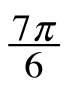radians to degrees

2.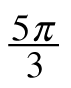radians to degrees

6.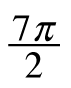radians to degrees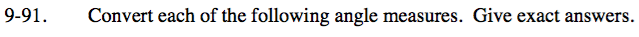There are multiple ways to approach these problems. Try different strategies to expand your personal toolkit.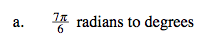Use the Hint from
problem 9-90.
Let π = 180° and simplify.
Notice, you do not need a calculator if you divide 180 by 6 as your first step.

210°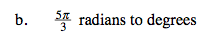Use the Hint or Help from problem 9-90.

$\text{Multiply } \frac{5 \pi}{3} \text{ by } \frac{180}{\pi}.$

Simplify by factoring out Giant Ones.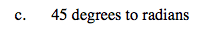Use the second part of the Help from problem 9-90.

$\frac{{\pi}}{4}$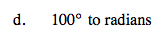Use the Help from problem 9-90 or try the More Help:

$\text{Use the proportion } \frac{\pi}{180°} = \frac{r}{100°}.$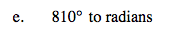See parts (c) and (d).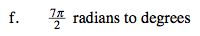See parts (a) and (b).

630°